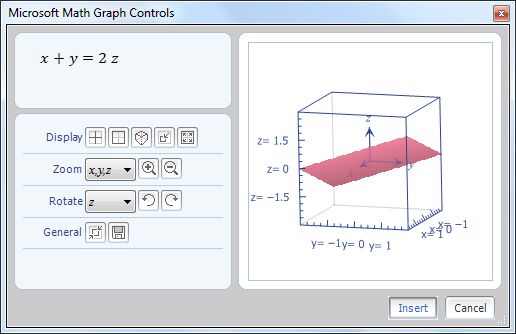Though Microsoft Office 2010 especially its Word software does come with a better way of inserting equation and other complicated mathematical symbols, sometimes you still face problems inserting complex equation as well as plotting graphs. So you need to install the Microsoft Mathematics add-in to ease your work.

### What can you do with Mathematics Add-in

• Compute standard mathematical functions such as roots and logarithms.
• Compute trigonometric functions such as sine and cosine.
• Find derivatives and integrals, limits, and sums and products of series.
• Perform matrix operations such as inverses, addition and multiplication.
• Perform operations on complex numbers.
• Plot 2-D graphs in Cartesian and polar coordinates.
• Plot 3-D graphs in Cartesian, cylindrical, and spherical coordinates.
• Solve equations and inequalities.
• Calculate statistical functions, such as mode and variance, on lists of numbers.
• Factor polynomials or integers.
• Simplify or expand algebraic expressions.### How to Install Mathematics Add-in

3. Then, start Word or OneNote program and you’ll see the new equation, computation and graphing options on the Mathematics tab.

•### Christine, August 27, 2010 @ 3:08 pmReply

Thanks for sharing KeeMan, I’ve tried.. it’s so handy.. ^^

•### Elisha Batuncang, August 27, 2010 @ 7:15 pmReply

Very useful piece of information. Lots of thanks to you. =)